# Quiz 10: Liabilities: Current, Installment Notes, and Contingencies

Company A is having retailing business that is working world wide. It is trading electronic and media product for consumers. Another company BB is also in same business. They are competitors. Difference is Company A is only trading through internet, whereas company BB is trading in both through trading and traditional way. a.Working Capital: It is the capital of the business which is used in day to day operations. It is a measure of a company's efficiency and financial position. Working capital is computed in two stages, when only current assets are cumulated are called as gross working capital and when total current assets are deducted from current liabilities is said to be net working capital. Following computation is given for computation of working capital. Prepare the net working Capital of both the company's as follows: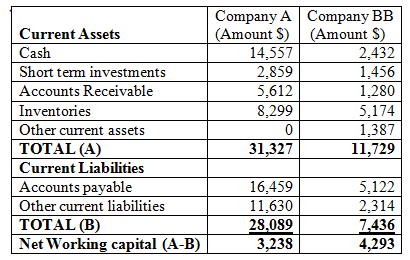Current assets are working assets which are working recurring in nature of company. Same is with current liabilities; this is because they are being used to compute working capital. Hence, working capital is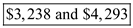of company A and BB respectively. B. Current ratio is nothing but division of current assets by current liabilities of the company. The current ratio is used to know financial position of company and investors use it to analyze the liquidity of a company and it also tells capability of company if it can pay its short term liabilities. Optimal current ratio is said to be two.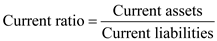Computation of current ratio for Company A is below;This current ratio is used to know that companies A have ability to repay its short-term liabi1ilities using its short-term assets. In this company does not seem to be optimal, as current assets are not even covering current liability. It gives an adverse reflection of operations done in company. Computation of current ratio for Company BB is below;In this company BB is closer to meet the optimal ratio (2:1) to know the company can repay his debt and payables using their cash, inventory and receivables.  Hence, Current ratio is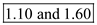of company A and BB respectively. C. Quick Ratio is current assets deducting inventory from all current assets divided by current liabilities. The quick ratio is a used to know company's immediate liquidity. It is famously know as acid test ratio in world of analysis.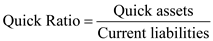For above calculation we need to compute quick assets of both the companies. Quick assets are computed when inventory is excluded from current assets. It is shown as-In this case only inventory is involved in quick assets calculation as prepaid expenses and any other item is not involved here. Now, Quick ratio will be computed on basis of above calculations;Optimal quick ratio is said to be one, so company A has quick ratio of 0.80 which is almost close to given thumb rule. Hence, company is in capability to pay off his debts.Company BB's quick ratio is seems to be more conservative than current ratio. Hence, Current ratio is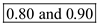of company A and BB respectively. d.The working capital can be usefully compared between two companies. The reason being both companies are related to same industry. And also they have same assets and working liabilities. Whereas, there is scale at which they are working is little different, one is more expanded then other is limited. Somehow, when whole financial statement will be seen and analyzed which includes fixed assets shareholders then overall position can be seen. As working capital ratio and quick ratio tells us financial position of companies. E. Best Buy Company has greater debt paying ability as current ratio is 1.60 as compared to Amazon Inc for which the same ratio is 1.10. F. Best Buy Company has greater short term debt paying ability and has more liquid funds. The quick ratio for Best Buy is 0.90 whereas for Amazon Inc it is 0.80. G. Following is a summary of above data which is accumulated for further analysis;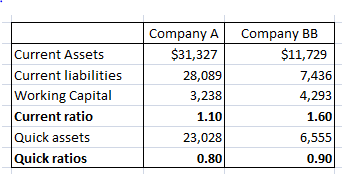Analysis of above data is given below; As it is seen that company A has more liabilities in working capital, as compare to company BB that is reason its current ratio is less then another. Current liabilities of company A are the one which is affecting its financial ratios. Even if quick assets are more then also this could be compensated. Quick ratio of both companies is near to optimal as current assets are reduced. In end, we can state that as company BB is more expanded and trading through two ways. Hence, their performance is seems to be better.
a.45-day notes Payable issued by B Company to T Bank for Face amount \$150,000 carrying interest rate of 10%. Since the note is not discounted hence the net proceeds will be \$150000. Hence, the Proceeds of note carrying 10% interest rate are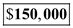. B. N company issued 45 days Note Payable at 10% discount. Its proceeds can be calculated as below: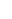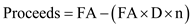Where FA is face amount, D is discount rate and n is time period.Given the FA of \$150,000, discount rate of 2% and time period of 45 days. Proceeds can be calculated as below.Hence, the Proceeds are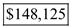when discounted at 10%